# Class 10 Science: CBSE Sample Question Paper (2020-21) - 4 Notes | Study CBSE Sample Papers For Class 10 - Class 10

## Class 10: Class 10 Science: CBSE Sample Question Paper (2020-21) - 4 Notes | Study CBSE Sample Papers For Class 10 - Class 10

The document Class 10 Science: CBSE Sample Question Paper (2020-21) - 4 Notes | Study CBSE Sample Papers For Class 10 - Class 10 is a part of the Class 10 Course CBSE Sample Papers For Class 10.
All you need of Class 10 at this link: Class 10

Class-X
Science Theory
TIME: 3 Hrs.
M.M: 80
General Instructions:
1. The question paper comprises four sections A, B, C and D. There are 36 questions in the question paper.
All questions are compulsory.
2. Section-A - Question no. 1 to 20 - all questions and parts thereof are of one mark each. These questions contain multiple-choice questions (MCQs), very short answer questions and assertion - reason type questions. Answers to these should be given in one word or one sentence.
3. Section-B - Question no. 21 to 26 are short answer type questions, carrying 2 marks each. Answers to these questions should be in the range of 30 to 50 words.
4. Section-C - Question no. 27 to 33 are short answer type questions, carrying 3 marks each. Answers to these questions should be in the range of 50 to 80 words.
5. Section-D - Question no. - 34 to 36 are long answer type questions carrying 5 marks each. Answers to these questions should be in the range of 80 to 120 words.
6. There is no overall choice. However, internal choices have been provided in some questions. A student has to attempt only one of the alternatives in such questions.
7. Wherever necessary, neat and properly labelled diagrams should be drawn.

SECTION A

Q.1. When hydrogen sulphide gas is passed through a blue solution of copper sulphate, a black precipitate of copper sulphide is obtained and the sulphuric acid so formed remains in the solution. The reaction is an example of a :     (1 Mark)
(a) Combination reaction
(b) Displacement reaction
(c) Decomposition reaction
(d) Double displacement reaction
Ans. d
Solution.
Double displacement reactions may be defined as the chemical reactions in which one component of each of both the reacting molecules is exchanged to form the products. During this reaction, the cations and anions of two different compounds switch places, forming two entirely different compounds.

Q.2. The chemical formula for plaster of Paris is:     (1 Mark)
(a) CaSO4 . 2H2
(b) CaSO4. H2O
(c) CaSO4. 1/2H2
(d) 2CaSO4 . H2O
Ans. c

Q.3. The laws of reflection hold true for: (1 Mark)
(a) plane mirrors only
(b) concave mirrors only
(c) convex mirrors only
(d) all reflecting surfaces
Ans. d

OR

When an object is kept within the focus of a concave mirror, an enlarged image is formed behind the mirror. This image is:     (1 Mark)
(a) real
(b) inverted
(c) virtual and inverted
(d) virtual and erect
Ans. d

Q.4. Name the functional group present in propanone.    (1 Mark)
Ans. Ketone

Q.5. State an important advantage of using alternating current (a.c.) over direct current (d.c.)     (1 Mark)
Ans. Advantage of AC: It can be transmitted over long distance without much loss of energy.

Q.6. Write the chemical name and chemical formula of the salt used to remove the permanent hardness of the water.    (1 Mark)
Ans. Sodium carbonate decahydrate, Na2CO3.10H2O

Q.7. How is the wall of the small intestine adapted for performing the function of absorption of food?    (1 Mark)
Ans. The inner lining of the small intestine has numerous finger-like projections called villi which increase the surface area for absorption.

OR

Out of a goat and a tiger, which one will have a longer small intestine? Justify your answer.
Ans. Goat because herbivores eating grass need a longer small intestine to allow the cellulose to be digested.

Q.8. Explain how ozone being a deadly poison can still perform an essential function for our environment.    (1 Mark)
Ans. The ozone layer protects us from the harmful effects of UV radiation.

Q.9. Give a reason why a food chain cannot have more than four trophic levels.    (1 Mark)

Ans. The loss of energy at each step is so great that very little usable energy remains after four trophic levels.

Q.10. For question numbers 10, two statements are given- one labelled Assertion (A) and the other labelled Reason (R). Select the correct answer to these questions from the codes (a), (b), (c) and (d) as given below :    (1 Mark)
Assertion: The food chain is responsible for the entry of harmful chemicals into our bodies.
Reason: The length and complexity of food chains vary greatly.
Options~
(a) Both A and R are true, and R is the correct explanation of the assertion.
(b) Both A and R are true, but R is not the correct explanation of the assertion.
(c) A is true, but R is false.
(d) A is false, but R is true.
Ans: (b) Both Assertion & Reasoning are correct, Reason is not the correct explanation of Assertion.

Q.11. For question numbers 11, two statements are given- one labelled Assertion (A) and the other labelled Reason (R). Select the correct answer to these questions from the codes (a), (b), (c) and (d) as given below :    (1 Mark)
Assertion: Greater number of individuals are present in lower trophic levels.
Reason: The flow of energy is unidirectional.
Options~
(a) Both A and R are true, and R is the correct explanation of the assertion.
(b) Both A and R are true, but R is not the correct explanation of the assertion.
(c) A is true, but R is false.
(d) A is false, but R is true.
Ans. (b) Both Assertion & Reasoning are correct, Reason is not the correct explanation of Assertion.

Q.12. List two biotic components of an ecosystem.    (1 Mark)
Ans. Plants, animals, micro-organisms.

OR

What is the food chain?
Ans. The food chain is a simple representation of energy flow in nature. It represents the series of organisms that eat other organisms any may in turn be eaten themselves.

Q.13. A student determines the focal length of a device ‘X’ by focusing the image of a distant object on a screen placed 20 cm from the device on the same side as the object. The device ‘X’ is    (1 Mark)
(a) Concave lens of focal length 10 cm
(b) Convex lens of focal length 20 cm
(c) Concave mirror of focal length 10 cm
(d) Concave mirror of focal length 20 cm
Ans: (d)
Solution.
Image formed by the concave mirror, in this case, is the same as when the object is at infinity. Due to the great distance, light rays will incident almost parallel to the principal axis. After reflection, all the rays will converge and meet at a principal focus. So, the focal length is 20 cm.

Q.14. Which of the following can make a parallel beam of light when light from a point source is incident on it?
(a) Concave mirror as well as a convex lens
(b) Convex mirror as well as a concave lens
(c) Two plane mirrors placed at 90° to each other
(d) Concave mirror as well as a concave lens
Ans. (a)
Solution.
When a point source of light is placed at the focus of the concave mirror then all light rays after reflection through the mirror will become parallel to the principal axis. When this point source of light is placed at the focus of the convex lens then after falling on the convex lens it will become parallel to the principal axis.

Q.15. Select the mismatched pair in the following and correct it.    (1 Mark)
(a) Bio-magnification — Accumulation of chemicals at the successive trophic levels of a food chain
(b) Ecosystem — Biotic components of the environment
(c) Aquarium — A man-made ecosystem
(d) Parasites — Organisms that obtain food from other living organisms
Ans. (b) Ecosystem- Biotic components of the environment
Solution.
Both biotic and abiotic components of the environment constitute an ecosystem.

Directions: Q. No 16-18 contain five sub-parts each. You are expected to answer any four subparts in these questions.
Q.16. Study the given experimental set-up and answer any four questions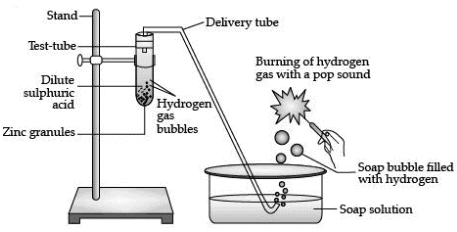(a) The above experimental set up shows a reaction between the metal and    (1 Mark)
(i) Acid
(ii) Metal carbonate
(iii) Metal hydrogen carbonate
(iv) Metal oxide
Ans: (i) It is a reaction between a metal (Zn) and an acid (H2SO4)

(b) Which gas is liberated during the process?    (1 Mark)
(i) Hydrogen gas
(ii) Carbon dioxide gas
(iii) Nitrogen gas
(iv) Hydrogen sulphide gas
Ans. (i) During the reaction between a metal and an acid, hydrogen gas is released.

(c) Identify the balanced chemical equation for the given reaction.    (1 Mark)
(i) Zn + H2SO4 → ZnSO4 + H2
(ii) 2Zn  +  2H2SO4 → 2ZnSO4 + 2H2
(iii) Zn + 2H2SO4 → 2ZnSO4 + H2
(iv) 2Zn  + H2SO4 → 2ZnSO4 + H2
Ans. (i) Zn + H2SO4 → ZnSO4 + H2

(d) What will happen if NaOH is used in place of dil. sulphuric acid and the test tube is heated?    (1 Mark)
(i) It will produce sodium zincate (salt) and hydrogen gas.
(ii) It will produce sodium sulphate and hydrogen gas.
(iii) It will produce sodium zincate (salt) and carbon dioxide gas.
(iv) It will produce sodium sulphate and carbon dioxide gas.
Ans. (i) It will produce sodium zincate (Salt) and hydrogen gas.
Zn (s) + 2NaOH (aq) → Na2ZnO2 (aq)  + H2 (g)

(e) How will you test for the gas evolved?    (1 Mark)
(i) Bring a glowing splint near the test tube, it will reignite.
(ii) Bring moist litmus paper is placed in a test tube of this gas, it turns red.
(iii) Bring a burning splint near the opening of a test tube containing this gas, a popping sound occurs.
(iv) Using universal litmus solution.
Ans: (iii) Test for H2 gas: The presence of H2 gas can be tested by passing the gas through soap solution and then bringing a burning splinter near the soap bubbles filled with the gas. If the gas burns with a pop sound, it is hydrogen.

A student fixes a sheet of white paper on a drawing board. He places a bar magnet in the centre of it. He sprinkles some iron filings uniformly around the bar magnet. Then he taps the board gently and observes that the iron filings arrange themselves in a particular pattern.
(a) Why do the iron filings arrange in a pattern?    (1 Mark)
(i) Due to force exerted by the magnet within its magnetic field.
(ii) Due to force exerted by the magnet outside the magnetic field.
(iii) Due to the pressure of the magnetic field.
(iv) Due to gravitational force.
Ans: (i) Due to the force exerted by the magnet within its magnetic field.

(b) The lines along which the iron filings align represent ___________. It moves from _________ .    (1 Mark)
(i) Gravitational force, North to South pole
(ii) Magnetic field lines, South to North pole
(iii) Gravitational force, South to North pole
(iv) Magnetic field lines, North to South pole
Ans: (iv) The lines represent magnetic field lines.

(c) What does the crowding of iron filings at the end of the magnet indicate?    (1 Mark)
(i) Magnetic field is weaker near the poles of the magnet.
(ii) Magnetic field is strongest near the poles of the magnet.
(iii) Magnetic field is neither weaker nor strongest near the poles of the magnet.
(iv) None of these
Ans: (ii) Crowding of iron filings at the ends of the magnet indicates that the magnetic field is strongest near the poles of the magnet.

(d) Which of the following statements is correct about magnetic field strength?    (1 Mark)
(i) Closer the lines, the more will be the strength and the farther the lines, the lesser will be the field strength.
(ii) Closer the lines, less will be the strength and farther the lines, more will be the field strength.
(iii) Magnetic field strength is the same everywhere.
(iv) None of these
Ans: (i) The strength of the magnetic field is indicated by the closeness of the field lines. The closer the lines, the more will be the strength and the farther the lines, the lesser will be the field strength.

(e) Which of these is not a source of the magnetic field?    (1 Mark)
(i) Magnet
(ii) Moving charges
(iii) Electric current
(iv) Battery
Ans: (iv) Battery is not a source of the magnetic field while another magnet, moving charges, and electric current are sources of the magnetic field.

In a thermite reaction, a compound of iron reacts with a metal.

(a) Which of the following metal is used in this reaction?    (1 Mark)
(i) Copper (Cu)
(ii) Aluminium (Al)
(iii) Magnesium (Mg)

(iv) Zinc (Zn)
Ans: (ii) Aluminium (Al)

(b) After completion of this reaction, a metal is obtained in the molten state. Identify the metal.    (1 Mark)
(i) Zinc (ii) Copper
(iii) Aluminium
(iv) Iron
Ans: (iv) Iron (Fe)

(c) The correct reaction in the form of a balanced chemical equation is:    (1 Mark)
(i) Fe2O3 + 2Al → 2Fe + Al2O3 + Heat.
(ii) Fe2O3 + Al → Fe + Al2O3 + Heat.
(iii) 2Fe2O3 + 2Al → 2Fe + 2Al2O3 + Heat.
(iv) 2Fe2O3 + Al → 2Fe + Al2O3 + Heat.
Ans: (i) Fe2O3 + 2Al → 2Fe + Al2O3 + Heat.

(d) Mention the most common use of this reaction is:    (1 Mark)
(i) In paints and varnishes
(ii) In the making of gold jewellery
(iii) To join railway tracks or cracked machine parts
(iv) In the heating element of electric geysers.
Ans: (iii) To join railway tracks or cracked machine parts.

(e) The reaction of metal with Al is ___________.    (1 Mark)
(i) Endothermic
(ii) Exothermic
(iii) Redox reaction
(iv) None of these
Ans: (ii) The reaction of metal with aluminium is exothermic.

Q.19. Commercial electric motors do not use :
(a) An electromagnet to rotate the armature
(b) Effectively large no. of turns of conducting wire in the current carrying coil
(c) A permanent magnet to crate the armature
(d) A soft iron core on which the coil is wound
Ans: c

Solution: Since permanent magnets do not produce strong magnetic fields it is not used in commercial electric motors.

Q.20. Three students A, B and C focused a distant building on a screen with the help of a concave mirror. To determine the focal length of the concave mirror they measured the distances as given below :    (1 Mark)
Student A: From mirror to the screen
Student B: From building to the screen
Student C: From building to the mirror
Who measured the focal length correctly?
(a) Only A
(b) Only B
(c) A and B
(d) B and C
Ans: (a) Image formed by the concave mirror, in this case, is the same as when the object is at infinity. Due to the great distance, light rays will incident almost parallel to the principal axis. After reflection, all the rays will converge and meet at the principal focus. A sharp image will be formed when the screen is closer to the mirror.

SECTION B

Q.21. (i) Define dispersion of light.
(ii) Name the colour that deviates least and the one which deviates most while
passing through a glass prism.    (2 Mark)
Ans: (i) Dispersion: Breaking up of white light into component colours.
(ii) Red colour deviates the least and violet deviates the most.

Q.22. 1 g of copper powder was taken in a China dish and heated. What change takes place on heating? When hydrogen is passed over this heated substance, a visible change is seen in it. Give the chemical equations of reactions.    (2 Mark)
Ans: The black colour substance formed by the reaction of copper with oxygen is Copper (II) oxide (CuO).
Chemical Reaction: 2Cu + O2 ➝ 2CuO
Hydrogen gas is passed over this heated material (CuO), the black coating on the surface turns brown as the reverse reaction takes place and copper is obtained.
CuO + H2 → Cu + H2O

Q.23. A student has two resistors - 2 Ω and 3 Ω. She has to put one of them in place of R2 as shown in the circuit. The current that she needs in the entire circuit is exactly 9A. Show by a calculation which of the two resistors she should choose.    (2 Mark)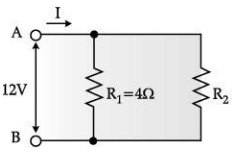Ans: The overall current needed = 9A.
The voltage is 12V Hence by ohm’s Law V = IR,
The resistance for the entire circuit = 12/9 =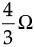= R
R1 and R2 are in parallel.
Hence, R =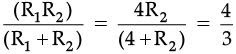R2 = 2Ω

Q.24. Give a test that can be used to confirm the presence of carbon in a compound. With a valency of 4, how is carbon able to attain noble gas configuration in its compounds?    (2 Mark)
Ans:
➤ Burn compound in air/ oxygen; Gas evolved turns lime water milky.
➤ By sharing its four valence electrons with other elements.

OR

The number of carbon compounds is more than those formed by all other elements put together. Justify the statement by giving two reasons.

Ans:

• Due to self linking ability of carbon/ catenation.
• Since carbon has a valency of four it can form bonds with four other atoms of carbon or atoms of some other monovalent element.
• Due to the small size of carbon it forms very strong and (or) stable bonds with other elements.

Q.25. The following observations were made by a student on treating four metals P, Q, R and S with the given salt solutions :    (2 Mark)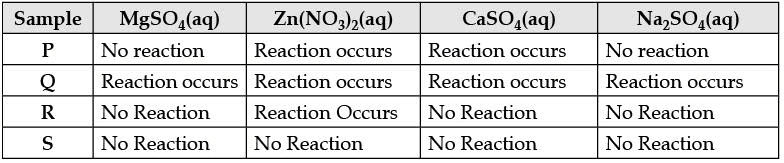Based on the above observations :
(a) Arrange the given samples in the increasing order of reactivity

(b) Write the chemical formulae of products formed when Q reacts with CuSO4 solution.
Ans: (a) S > R > P > Q

(b) Cu and QSO4

Q.26. (A) Draw the pattern of magnetic field lines through a circular loop:
mark the direction of:     (2 Mark)

(i) the electric current in the loop
(ii) magnetic field lines.
(B) Two circular coils A and B are placed close to each other. If the current in coil A is changed, will some current be induced in coil B? Give reason.
Ans: (A)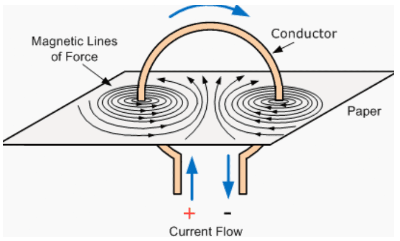(B) Current will be induced in coil B. When the current in coil A is changed, the Magnetic field associated with the coil also changes. As coil B is placed close to A, thus magnetic field lines around this coil also change. Due to the change in magnetic field lines associated with coil B, a current is also induced in it.

Section C

Q.27. In humans, there is a 50% probability of the birth of a boy and a 50 % probability that a girl will be born. Justify the statement on the basis of the mechanism of sex-determination in human beings.

Ans: In human beings, the genes inherited from our parents decide whether it will be a boy or girl. Women have a perfect pair of sex chromosomes (XX). But, men have a mismatched pair (XY).
All children will inherit an X chromosome from their mother regardless of whether they are boys or girls. Thus, the sex of the children will be determined by what they inherit from their father. A child who inherits an X chromosome from her father will be a girl, and one who inherits a Y chromosome from him will be a boy.

Q.28. (a) Plastic cups were used to serve tea in trains in the early days- these could be returned to the vendors, cleaned and reused. Later, Kulhads were used instead of plastic cups. Now, paper cups are used for serving tea.    (1 Mark)

Ans: The use of plastic cups raised the concern towards hygiene thus they were replaced by disposable plastic cups.

(b) What are the reasons for the shift from Plastic to Kulhads and then finally to paper cups?    (2 Mark)
Ans: Plastic cups are non-biodegradable and harm the environment. They were, thus, replaced by Kulhads. Making Kulhad, which is made of clay on a large scale resulted in the loss of top fertile soil.
Now, disposable paper cups are used because the paper can be recycled, it is biodegradable and is eco-friendly material, which does not cause environmental pollution.

Q.29. The Refractive index of water with respect to air is 1.33 and that of a diamond is 2.42.    (3 Mark)
(i) In which medium does the light move faster, water or diamond?
(ii) What is the refractive index of diamond with respect to water?
Ans: (i) Refractive index = speed of light in vacuum/speed of light in the medium.

Since the refractive index of a diamond is more, hence the speed of light is lesser in a diamond.
(ii) Let the speed of light in water be vw and in diamond be vd.
Refractive index of diamond w.r.t water is said to be: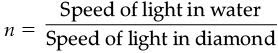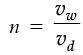Dividing both numerator and denominator by speed of light [c] we get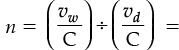The inverse ratio of the refractive index of water and diamond.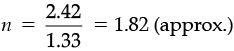Q.30. Write the names given to the vertical columns and horizontal rows in the Modern Periodic Table. How does the metallic character of elements vary on moving down a vertical column? How does the size of the atomic radius vary on moving left to right in a horizontal row? Give reason in support of your answer in the above two cases.    (3 Mark)

Ans: Vertical Column — Groups

Horizontal Rows — Periods

(i) Metallic character increases.

Reason: The ability to lose electrons increases on moving down the group due to the increase in distance between the nucleus and the valence electrons/decrease in the attraction between the nucleus and the valence electrons.

Reason: The nuclear charge increases on moving from left to right across a period resulting in an increase in the attraction between the nucleus and the valence electrons.

Q.31. State the cause of dispersion of white light passing through a glass prism. How did Newton show that the white light of the sun contains seven colours using two identical glass prisms? Draw a ray diagram to show the path of light when two identical glass prisms are arranged together in an inverted position with respect to each other and a narrow beam of white light is allowed to fall obliquely on one of the focus of the first prism.    (3 Mark)

Ans: Cause of dispersion of white light: Different colours of light bend through different angles with respect to the incident ray as they pass through a prism.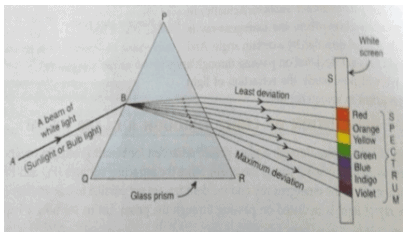OR

Define angle of deviation. Why do different components of white light split up into spectrum when it passes through a triangular glass prism? Show the angle of deviation for red colour when white light passes through a prism.

Ans: The angle between the extended incident ray and the emergent ray is called the angle of deviation. This is because the different colours travel through a glass prism at different speeds.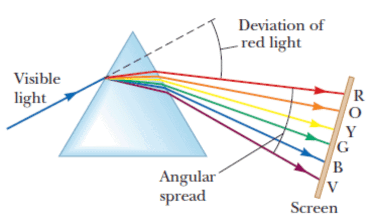Q.32. What is a homologous series of carbon compounds? List its any two characteristics. Write the name and formula of the next higher homologous HCOOH.    (3 Mark)

Ans: (a) A group of organic compounds having the same functional group and similar structures in which any two successive members differ by – CH2.
(b) (i) All members have similar chemical properties
(ii) There is gradation in the physical properties. (or any other)
(c) Name — Ethanoic acid/Acetic acid
Formula — CH3COOH

Q.33. A variegated leaf with green and yellow patches is used for an experiment to prove that chlorophyll is required for photosynthesis. Before the experiment, the green portions (A), and the pale yellow portions (B), are observed. What will be the colour of ‘A’ just before and after the starch test? Also write the equation of photosynthesis and mark, as well as validate, from which molecule the byproduct is obtained?    (3 Mark)
Ans: Just before Starch test — Pale yellow

Just after the Starch test – Blue black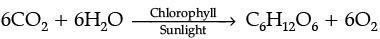O2 is obtained from water (H2O), as the splitting of water results in the formation of hydrogen (used for making glucose) and oxygen (by-product).

SECTION  D

Q.34. (a) What is the law of dominance of traits? Explain with an example.

(b) Why are the traits acquired during the lifetime of an individual not inherited? Explain.    (5 Mark)

Ans: (a) Law of the dominance of traits: It states that “When parents having pure contrasting characters are crossed then only one character expresses itself in F1 generation. This character is the dominant character and the character which cannot express itself is called the recessive character”.
Let us take an example of tall and dwarf in the pea plant. When pure line tall (TT) plants were crossed with pure line dwarf (tt) plants, offspring were all heterozygous tall (Tt). The appearance of all Tall plants in the F1 generation shows that tallness is the dominant character while dwarfness is the recessive character. The ratio of Tall to Dwarf in the F2  generation is 3:1.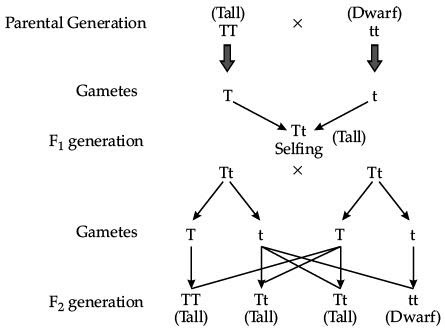(b) Characters that a person acquires during one’s lifetime are known as acquired characters/traits.
Such changes do not occur in the reproductive tissues. Changes in the non-reproductive tissues are not passed on to the DNA of the germ cells and therefore not inherited by the next generation.

Q.35. Draw a ray diagram in each of the following cases to show the formation of the image, when the object is placed :    (5 Mark)

(i) between the optical centre and principal focus of a convex lens.

(ii) anywhere in front of a concave lens.

(iii) at 2F of a convex lens.

State the signs and values of magnifications in the above-mentioned cases (i) and (ii).

Ans: (i) When an object is placed between the optical centre and principal focus of a convex lens: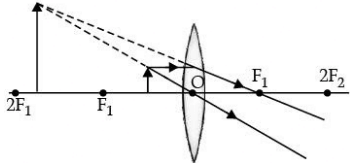The image formed is virtual and erect, so the sign of magnification will be positive. Also, since, an image formed is magnified, so the value of magnification will be greater than one.
(ii) When an object is placed anywhere in front of a concave lens: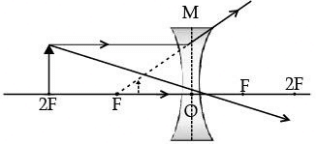The image formed is virtual and erect, so the sign of magnification will be positive. Also, since, an image formed is diminished, so the value of magnification will be less than one.
(iii) When an object is placed at 2F of a convex lens: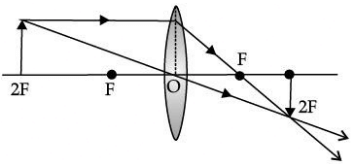OR

An object 4.0 cm in size, is placed 25.0 cm in front of a concave mirror of focal length 15.0 cm.
(i) At what distance from the mirror should a screen be placed in order to obtain a sharp image?

(ii) Find the size of the image.

(iii) Draw a ray diagram to show the formation of an image in this case.

Ans: Given, Height of object (h0) = 4 cm

Object distance (u) = -25 cm

Focal length (concave mirror) (f) = -15 cm

(i) By applying the mirror formula,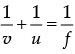Or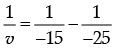Or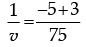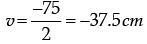The negative sign indicates that the image is formed in front of the mirror. Therefore, the screen must be placed in front at a distance of 37.5 cm.

(ii) As per magnification formula,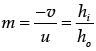Or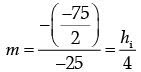Or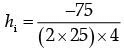h1 = - 6m

The negative sign indicates that the image is below the principal axis.  Therefore, the size of the image is 6 cm.

(iii) Ray diagram :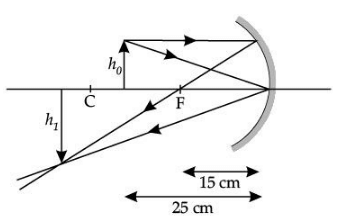Q.36. (a) A gas is released during photosynthesis. Name the gas and also state the way by which the gas is evolved.
(b) What are stomata? What governs the opening and closing of stomata?     (5 Mark)
Ans: (a) The gas released during the process of photosynthesis is oxygen. Oxygen liberated during photosynthesis comes from water. During photosynthesis, plants absorb carbon dioxide and sunlight to produce carbohydrates. The solar energy trapped by chlorophyll breaks down water molecules by the process of photolysis. Photolysis of water releases oxygen. This released oxygen gets emitted in the atmosphere.

(b) Stomata are tiny pores present on the surface of the leaves.

The opening and closing of stomatal pores are controlled by the turgidity of guard cells. When guard cells uptake water from surrounding cells, they swell to become a turgid body. This enlarges the pore in between and causing stomatal opening.

When water is released, they become flaccid. This closes the pore in between causing stomatal closing.

OR

(a) Draw a diagram of the human alimentary canal and label - gall bladder, pancreas, liver and small intestine on it.
(b) Give two reasons to explain why the absorption of digested food occurs mainly in the small intestine.
Ans: (a)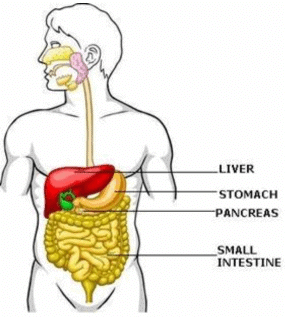(b) Absorption of digested food occurs mainly in the small intestine because it has finger-like projections called villi which help in the absorption of food into blood. Villus contains a lymph capillary called lacteal in the centre. Lacteal in turn is surrounded by a network of thin and small blood vessels called blood capillaries close to its surface. As the food moves slowly between, over and around the villi, the surface of the villi absorbs the digested food materials into the blood flowing through them. Blood, in turn, carries the absorbed food materials to all the parts of the body. In the cells, food is used for energy, repair and growth. The process is known as assimilation.

The document Class 10 Science: CBSE Sample Question Paper (2020-21) - 4 Notes | Study CBSE Sample Papers For Class 10 - Class 10 is a part of the Class 10 Course CBSE Sample Papers For Class 10.
All you need of Class 10 at this link: Class 10Use Code STAYHOME200 and get INR 200 additional OFF

## CBSE Sample Papers For Class 10

174 docs|7 tests

Track your progress, build streaks, highlight & save important lessons and more!

,

,

,

,

,

,

,

,

,

,

,

,

,

,

,

,

,

,

,

,

,

;